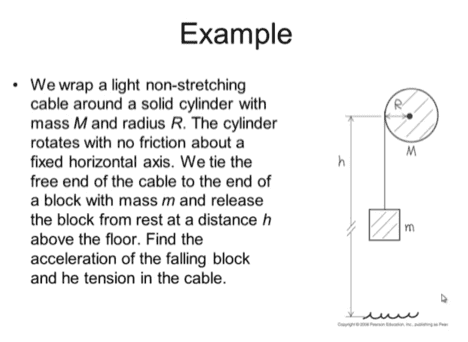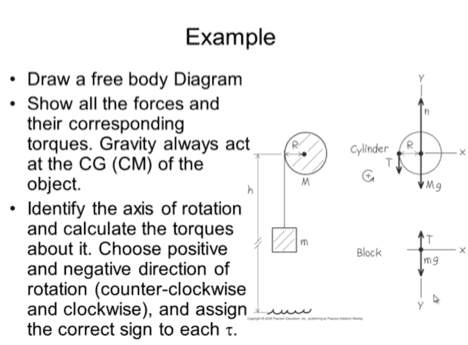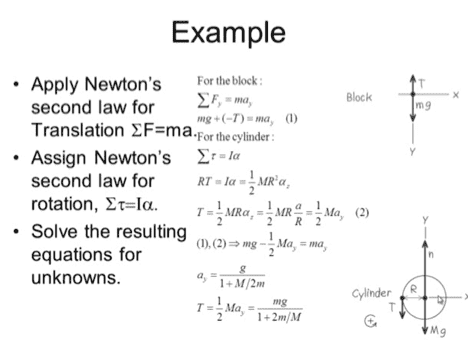# Angular Acceleration

## Homework StatementThis is not really a question on how to solve the problem, I'm just trying to get clarification on something. For angular acceleration, α, can someone explain to me what αz is? And why does αz = α / R = αy? I understand the rest of the problem, I just don't understand where or what αz is and how αz = α / R = αy. Thanks in advance for any help given!

TSny
Homework Helper
Gold Member
For angular acceleration, α, can someone explain to me what αz is?
##\alpha_z## apparently represents the angular acceleration for rotation about the z-axis.
And why does αz = α / R = αy?
The print in the picture is small and hard to read. In equation (2) I think it states that ## \alpha_z = \large \frac{a}{R}##, not ##\alpha_z = \large \frac{\alpha}{R}.##

##\alpha_z## apparently represents the angular acceleration for rotation about the z-axis.

The print in the picture is small and hard to read. In equation (2) I think it states that ## \alpha_z = \large \frac{a}{R}##, not ##\alpha_z = \large \frac{\alpha}{R}.##

Ohhh, you're right about the a/R not α/R. But can you explain to me why does a/R = ay?

TSny
Homework Helper
Gold Member
Ohhh, you're right about the a/R not α/R. But can you explain to me why does a/R = ay?
They aren't claiming that a/R = ay.

The R cancels: (1/2)MR(a/R) = (1/2)May. The "a" on the left is the same as ay.

They aren't claiming that a/R = ay.

The R cancels: (1/2)MR(a/R) = (1/2)May. The "a" on the left is the same as ay.

Ok I see. And just to clarify, if I divide a linear acceleration by the radius R then we get angular acceleration?

Nvm! Figured it out. Thank yoooou! :)

Last edited: